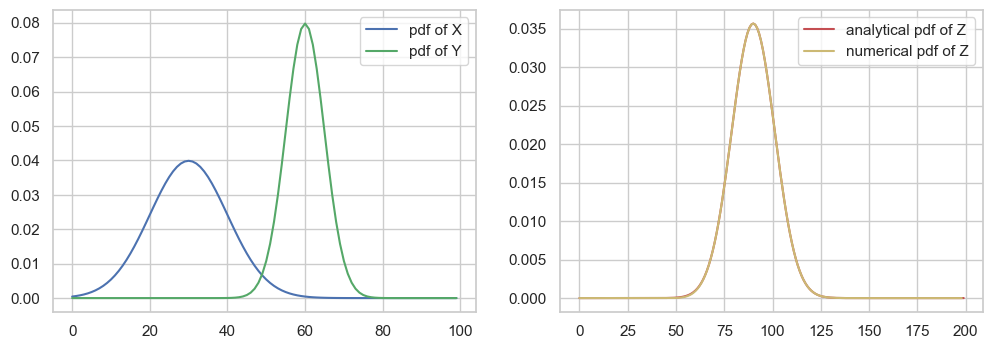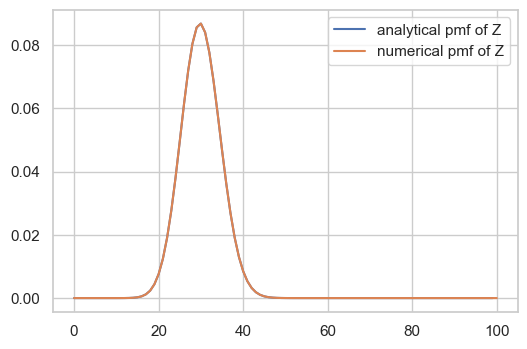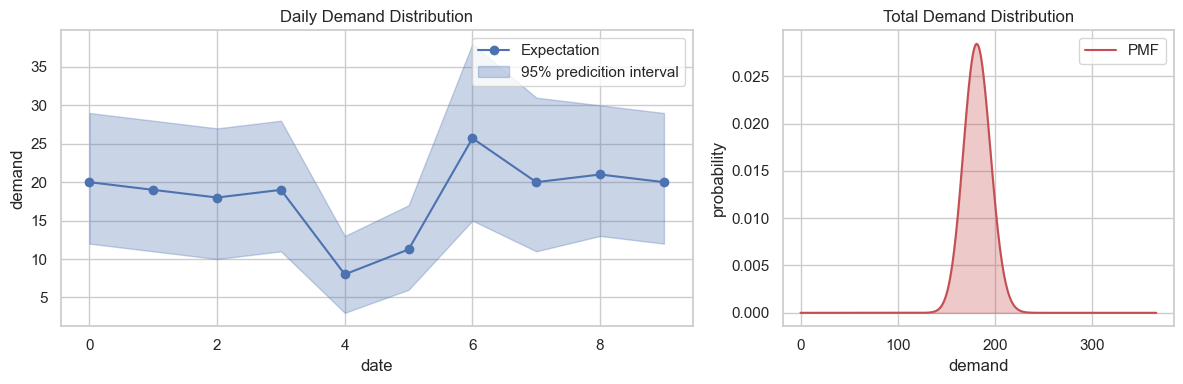## 方法

$S_n=\sum_{i=1}^{n} X_i$

$S_n = S_{n-1} + X_n$

• $X$$Y$ 是离散型随机变量，则 $Z$ 的概率质量函数为 $X$ 的概率质量函数与 $Y$ 的概率质量函数的离散卷积：

$P(Z=z) = \sum_{k=-\infty}^{+\infty}P(X=k)\cdot P(Y=z-k)$

• $X$$Y$ 是连续型随机变量，则 $Z$ 的概率密度函数为 $X$ 的概率密度函数与 $Y$ 的概率密度函数的卷积：

$f_Z(z) = \int_{-\infty}^{+\infty}f_X(x)f_Y(z-x)\mathrm dx\equiv f_X*f_Y$

## 例子## 附录

$\hat f(\omega) = \int_{-\infty}^{+\infty}f(t)\mathrm e^{-i\omega t}\mathrm dt$

$\hat g(\omega) = \int_{-\infty}^{+\infty}g(t)\mathrm e^{-i\omega t}\mathrm dt$

$h(t)$ 的傅里叶变换为

\begin{aligned} \hat h(\omega) &= \int_{-\infty}^{+\infty}h(t)\mathrm e^{-i\omega t}\mathrm dt\\ &= \int_{-\infty}^{+\infty}\left[\int_{-\infty}^{+\infty}f(\tau)g(t-\tau)\mathrm d\tau\right]\mathrm e^{-i\omega t}\mathrm dt\\ &= \int_{-\infty}^{+\infty}\int_{-\infty}^{+\infty}f(\tau)g(t-\tau)e^{-i\omega t}\mathrm dt\mathrm d\tau \end{aligned}

$s=t-\tau$，则 $\mathrm ds = \mathrm dt$

\begin{aligned} \hat h(\omega) & = \int_{-\infty}^{+\infty}\int_{-\infty}^{+\infty}f(\tau)g(s)e^{-i\omega (\tau+s)}\mathrm ds\mathrm d\tau\\ &= \int_{-\infty}^{+\infty}f(\tau)e^{-i\omega \tau}\mathrm d\tau\int_{-\infty}^{+\infty}g(s)e^{-i\omega s}\mathrm ds\\ &=\hat f(\omega)\cdot\hat g(\omega) \end{aligned}